Like   Tweet   Pin   +1   in/* styles */ Wellbeing + Performance Stories, Tips and Resources   ||   Issue #27 June 2020
 table div table+table+table div table{width:100%;padding:0}table div table+table+table div table img{width:96.23%;padding:0;float:none}table div table+table+table div table td{width:100%;padding:0 1.88% 18px}/* styles */In this issue I talk about the 'void', which is important for achieving goals. There's also a Tai Chi tip as well as two You tube clips on Chi Flow and Tai Chi walking. I've included a link to an article that provides five tips for peak performance and, as usual, there's an insightful Lao Tzu quote.

I hope you enjoy the read.

Regards
Chris Bennett
Chris Chi

 table div table+table+table+table+table div table{width:100%;padding:0}table div table+table+table+table+table div table img{width:96.23%;padding:0;float:none}table div table+table+table+table+table div table td{width:100%;padding:0 1.88% 18px}/* styles */## Don't Avoid the Void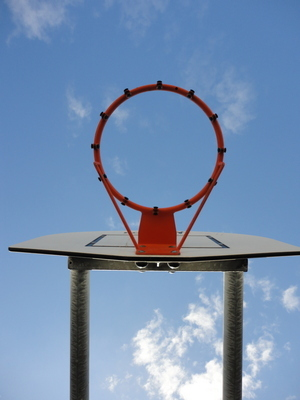The term 'void' in Tai Chi means nothingness and this is a necessary state if you are to achieve your goals with as little effort as possible.

This concept of the 'void' can be applied to any human activity. In this post I'll discuss it in terms of sport - in particular, the free throw in basketball.

 table div table+table+table+table+table+table+table+table div table{width:100%;padding:0}table div table+table+table+table+table+table+table+table div table img{width:96.23%;padding:0;float:none}table div table+table+table+table+table+table+table+table div table td{width:100%;padding:0 1.88% 18px}/* styles */## Tai Chi Tip

 /* styles */ Training - Focus on quality of repetitions not just quantity.
 table div table+table+table+table+table+table+table+table+table+table+table div table{width:100%;padding:0}table div table+table+table+table+table+table+table+table+table+table+table div table img{width:96.23%;padding:0;float:none}table div table+table+table+table+table+table+table+table+table+table+table div table td{width:100%;padding:0 1.88% 18px}/* styles */## Improve the Flow of Chi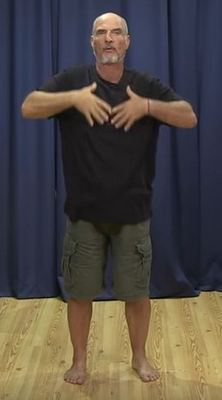In this 3.27 clip Bruce Frantzis, from Energy Arts, discusses how to get your Chi to flow though your body.

He covers how to 'release your upper body strength, soften your breath and sink the mind down to the lower tan tien'.

Good clear tips on how to relax your mind and body , which is necessary to perform any activity.

 table div table+table+table+table+table+table+table+table+table+table+table+table+table+table div table{width:100%;padding:0}table div table+table+table+table+table+table+table+table+table+table+table+table+table+table div table img{width:96.23%;padding:0;float:none}table div table+table+table+table+table+table+table+table+table+table+table+table+table+table div table td{width:100%;padding:0 1.88% 18px}/* styles */## Tai Chi Walking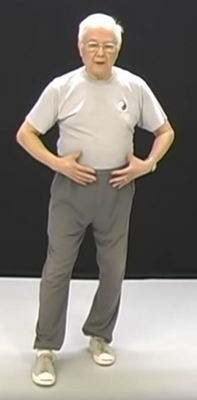Dr. Stephen Hwa gives a short detailed explanation on how to step in Tai Chi in this 5.45 clip.

He covers footwork technique and also the role of internal energy flow with regards to footwork.

There is another method of Tai Chi walking skills I use when I teach students, but this is a good clip for beginners.

 table div table+table+table+table+table+table+table+table+table+table+table+table+table+table+table+table+table div table{width:100%;padding:0}table div table+table+table+table+table+table+table+table+table+table+table+table+table+table+table+table+table div table img{width:96.23%;padding:0;float:none}table div table+table+table+table+table+table+table+table+table+table+table+table+table+table+table+table+table div table td{width:100%;padding:0 1.88% 18px}/* styles */## 5 Peak Performance Tips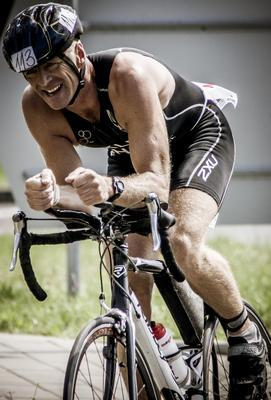Elizabeth Quinn's handy article on 5 steps to reach your athletic peak performance.

She covers sports psychology, nutrition/hydration, fitness testing/assessment, safety and injury prevention, shoes and feet.

(Photo courtesy of Gabor from Hungary via Morguefile)

 table div table+table+table+table+table+table+table+table+table+table+table+table+table+table+table+table+table+table+table+table div table{width:100%;padding:0}table div table+table+table+table+table+table+table+table+table+table+table+table+table+table+table+table+table+table+table+table div table img{width:96.23%;padding:0;float:none}table div table+table+table+table+table+table+table+table+table+table+table+table+table+table+table+table+table+table+table+table div table td{width:100%;padding:0 1.88% 18px}/* styles */## Latest NewsSince the coronas virus pandemic hit, all my face to face workshops for residents and staff at aged care centres have stopped and I've used the time available to work on a new online course for sports coaches.

I've drawn ideas from my face to face Sports Chi for players workshops and my background in coaching basketball. To find out more about my approach to teaching coaches and players, check out my Sports Chi flyer.

(Photo courtesy of JPPI via Morguefile)

 table div table+table+table+table+table+table+table+table+table+table+table+table+table+table+table+table+table+table+table+table+table+table+table div table{width:100%;padding:0}table div table+table+table+table+table+table+table+table+table+table+table+table+table+table+table+table+table+table+table+table+table+table+table div table img{width:96.23%;padding:0;float:none}table div table+table+table+table+table+table+table+table+table+table+table+table+table+table+table+table+table+table+table+table+table+table+table div table td{width:100%;padding:0 1.88% 18px}/* styles */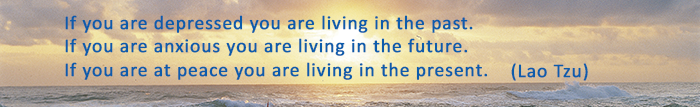table div table+table+table+table+table+table+table+table+table+table+table+table+table+table+table+table+table+table+table+table+table+table+table+table+table div table{width:100%;padding:0}table div table+table+table+table+table+table+table+table+table+table+table+table+table+table+table+table+table+table+table+table+table+table+table+table+table div table img{width:96.23%;padding:0;float:none}table div table+table+table+table+table+table+table+table+table+table+table+table+table+table+table+table+table+table+table+table+table+table+table+table+table div table td{width:100%;padding:0 1.88% 18px}/* styles *//* styles */
 table div table+table+table+table+table+table+table+table+table+table+table+table+table+table+table+table+table+table+table+table+table+table+table+table+table+table+table div table{width:100%;padding:0}table div table+table+table+table+table+table+table+table+table+table+table+table+table+table+table+table+table+table+table+table+table+table+table+table+table+table+table div table img{width:96.23%;padding:0;float:none}table div table+table+table+table+table+table+table+table+table+table+table+table+table+table+table+table+table+table+table+table+table+table+table+table+table+table+table div table td{width:100%;padding:0 1.88% 18px}/* styles *//* styles */